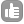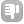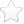2Are there any modern programs (preferably freeware) that will simulate line broadening in the presence of exchange. Somewhere I have a copy of "DNMR3" (on a 5 1/4 " floppy) and I feel it will be a nightmare trying to get this to work (particularly the graphics output). asked Sep 18 '09 at 07:16Evgeny Fadeev 577●1 These matlab scripts are based on Prof. Alex Bain's MEXICO program... https://docs.google.com/file/d/0B4NAn8pA4nzPQW0zTUhBeUFIYXc/edit?usp=sharing - Jana (Apr 15 '13 at 15:53) For QM based chemical exchange simulation you can try 'Spinach' by Prof. Ilya Kuprov. http://spindynamics.org/Spinach.php - Jana (Apr 19 '13 at 21:47)1The SpinWorks program has a graphical interface to both DNMR3 and MEXICO. Modified compiled versions of DNMR3 and MEXICO are both included with SpinWorks. It will run on everything from Windows 2000 to Windows 7, and will run on Macs under Parallels or Bootcamp. -Kirk answered Dec 19 '09 at 07:40Kirk Marat 7110The two programs I know are lineshapekin by Prof. Evgenii Kovrigin and MEXICO by Prof. Alex Bain. answered Sep 18 '09 at 10:02Evgeny Fadeev 577●10Thanks. The MEXICO program looks good, but it only seems to support windows NT (not XP or vista). answered Sep 22 '09 at 06:42Hi Dstephenson, Mexico is an excellent program for Chemical exchange simulation, likewise, Lineshapekin is very comprehensive with lots of implemented complex mechanisms for line shape analysis. For understanding purpose, here is the matlab version of 1D chemical exchange simulation based on Prof. Alex Bain's work.

## The following program requires another m-script "poplig", which is included at the end

``` % Simulation of 1D NMR with chemical exchange using Bloch-McConnell equations for a two-state ```

``` clc; % Parameters PI=22/7; % Larmor frequency W_1 = 7; % ppm W_2 = 8; % ppm O_1 = 5; % ppm %Plot parameter W_limit=1; % +/- 1ppm W_resol=0.01; Wupper=max(W1,W_2)+1; Wlower=min(W1,W_2)-1; Wpt=(Wupper-Wlower)/Wresol; % Relaxation rate R_1 = 0.05; % ppm R_2 = 0.05; % ppm % Kinetic rates k1 = 1000; %Hz k_1 = 100; %Hz dimension=2; % Scale S_1=1; S_2=1; % Spectrometer field MHz = 500; % MHz % Titration parameters L_T=[0 1 10 30 70 100 200 300 500 700 1000]; % mM P_T=0.22; % mM ligcount = size(LT); % Calculation of free ligand L=zeros(size(L_T)); P=zeros(size(L_T)); PL1=zeros(size(LT)); pop=zeros(size(L_T),dimension); for i=1:lig_count(2) x0=0.5; Total_L=L_T(i)*0.001; Total_P=P_T*0.001; % Population & Free ligand calculation function options=optimset('MaxIter',1000,'MaxFunEvals',1000); S=@(x)poplig(x,Total_L,Total_P,k1,k_1); [O,fval]=fsolve(S,x0,options); P(i)=max(O)*Total_P; PL_1(i)=Total_P-P(i); L(i)=Total_L-PL_1(i); pop(i,1)=max(O); pop(i,2)=1-max(O); end % Setting the Larmor, Relax and kinetic matrices Larmor=[2PIW1*MHz*1i 0; 0 2*PI*W2MHz1i]; Relax=[PIR_1MHz 0; 0 PIR_2MHz]; Comp=zeros(dimension); V_col=zeros(dimension); V1row=zeros(dimension); Pop_col=zeros(dimension); Intensity=zeros(dimension); r=size(L_T); t=round(W_pt); Spectrum_comp=zeros(t,dimension*r(2)); Spectrum=zeros(t+1,r(2)); for i=1:lig_count(2) Kinetic=[-k1L(i) k_1; k1L(i) -k_1]; Liouville=(Larmor+Relax-Kinetic); [V,U]=eig(Liouville); V_1=inv(V); % Calculating the individual components Vcolsum=sum(V(:,1:end),1); %for j=1:dimension % V1row(j)=V(j,:); %end Pop_col=pop(i,:); Popcol=Popcol'; for k=1:dimension Intensity(k)=Vcolsum(k)(V_1(k,:)Pop_col); end % Calculation of the line shape from the intensities for k=1:dimension a=real(Intensity(k)); b=imag(Intensity(k)); c=real(U(k,k)); d=imag(U(k,k)); for j=1:t omegav = (W_lower+(j-1)*W_resol) * MHz * 2 * 22/7; % PPM to HZ and then to angular freq % denom = (c * c) + ((d - omegav) * (d - omegav)); % num = ((a * c) + b * (d - omegav)); % Juz altering the sign of abs vs dispersive compared to the derivation % Spectrum_comp(j,(i-1)*dimension+k) = (num / denom); num=real((a+ b*1i)/(c+(d-omegav)*1i)); Spectrum_comp(j,(i-1)*dimension+k) = num; Spectrum(j,i) = Spectrum(j,i) + Spectrum_comp(j,(i-1)*dimension+k); end % components end % dimension end % lig_count % Plotting the spectra figure; plot(Spectrum(1:Wpt,1:ligcount(2))); poplig.m function F=poplig(x,TotalL,TotalP,k1,k_1) F=-k1(Total_L-Total_P+x(1)TotalP)*x(1)+k1*(1-x(1)); ```

answered Apr 09 '13 at 22:08https://docs.google.com/file/d/0B4NAn8pA4nzPQW0zTUhBeUFIYXc/edit?usp=sharing - Jana (Apr 15 '13 at 15:44)